# VLOOKUP and HLOOKUP combined Excel formula (with example)

In this article, we will see how VLOOKUP and HLOOKUP Combined functions work together in Excel formulas. Here we will use VLOOKUP and HLOOKUP combinedly to solve a problem. The basics of VLOOKUP and HLOOKUP will be shown. We will be using an example to find out a specific result using the combination of VLOOKUP and HLOOKUP.

## Basics of VLOOKUP and HLOOKUP

Before solving the problem using combined VLOOKUP and HLOOKUP, let`s get some basic idea about the functions.

### VLOOKUP Description

When you want to look up for a value vertically in Excel you need to use the VLOOKUP function. It is a built-in function in Excel which is under the Lookup function category.

### Syntax

=VLOOKUP (Lookup_Value, Table_Range, Column_Index, [Range_Lookup])

### Arguments

Lookup_Value – the value which you will look up vertically from the first column of the Table_Range.

Table_Range– Defines the range of the VLOOKUP function.

Column_Index- The column number from the Table_Range by which the matched value will be returned.

Range_Lookup– It`s an optional argument. Write False to get Exact Match and True for an approximate match. True is the default parameter while omitting this argument.

### Example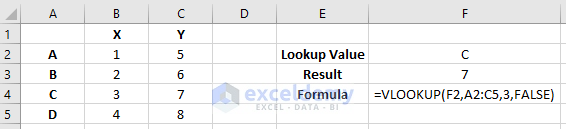### HLOOKUP Description

When you want to look up for a value horizontally in Excel you need to use the HLOOKUP function. It is also a built-in function in Excel which is under the Lookup function category.

### Syntax

=HLOOKUP (Lookup_Value, Table_Range, Row_Index, [Range_Lookup])

### Arguments

Lookup_Value– Value which will be found out horizontally from the first row of the Table_Range.

Table_Range– Defines the range of the HLOOKUP function.

Row_Index– The row number from the Table_Range by which the matched value will be returned.

Range_Lookup– It`s an optional argument. Write False to get Exact Match and True for an approximate match. True is the default parameter while omitting this argument.

### Example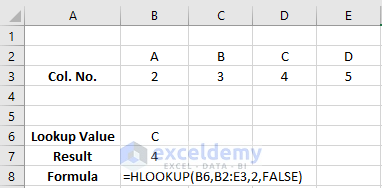## VLOOKUP & HLOOKUP Combined Together in an Excel Formula

Let`s look into an example where different products name with its monthly sales is given. We want to insert a formula in the worksheet where, by indicating the product and months name, the formula will give us the desired result.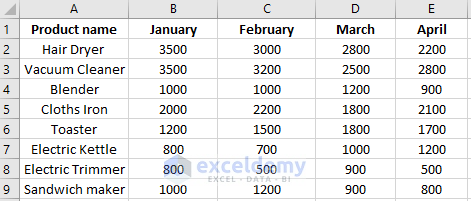For finding the result. Here we will be using the VLOOKUP and HLOOKUP functions together. Firstly, let`s look into the HLOOKUP function. In a different place of your worksheet insert months name along with the column number. By using the HLOOKUP function we will find the Column number first. In cell H6, write down the formula,`=HLOOKUP(H5,B14:E15,2,FALSE)` It will look for the month name in the range B14: E15. The row index is given here as 2. So, the formula will return the value 5.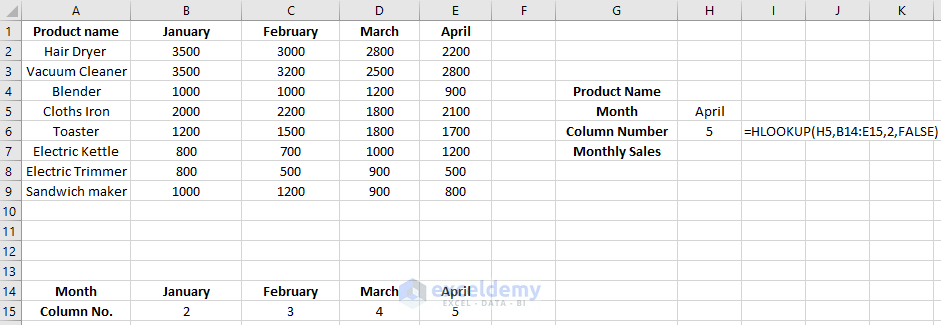Click to see the full image

Now, we will combine the HLOOKUP function with the VLOOKUP function to find the monthly sales of a product. In cell H7, write down the formula `=VLOOKUP(H4,A2:E9,HLOOKUP(H5,B14:E15,2,FALSE), FALSE) `and press Enter. It will evaluate the result that we wanted. As we indicate Product name Toaster and Month name April. So, it will give us the result 1700 in cell H7. By changing the names in cell H4 and H5 we will get different results.

Here the formula, `=VLOOKUP(H4,A2:E9,HLOOKUP(H5,B14:E15,2,FALSE), FALSE) `lookup for the value Toaster which is in cell H4 in the range A2: E9. In the function of VLOOKUP, the formula `HLOOKUP(H5,B14:E15,2,FALSE) `is given as the Column_Index. So instead of defining Column_Index with numbers, you are doing this with the HLOOKUP function. The False arguments will give you the exact match.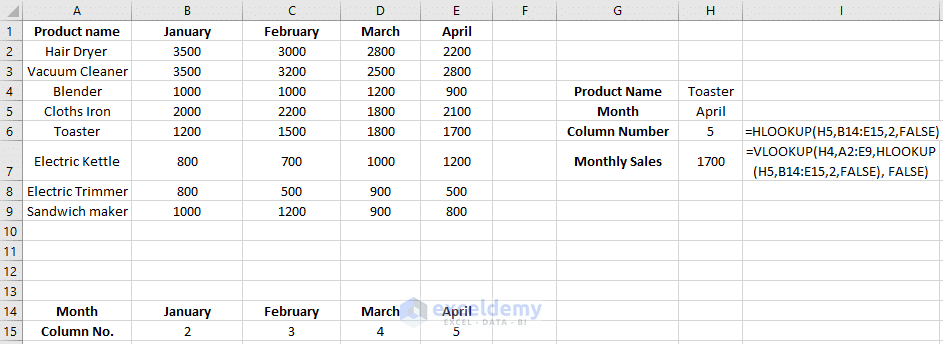Click to see the full image

Let`s check this formula for a different product and month. Now we will choose the product Blender and month February to find the monthly sales of the month February. In cell H4 and H5, write Blender and February.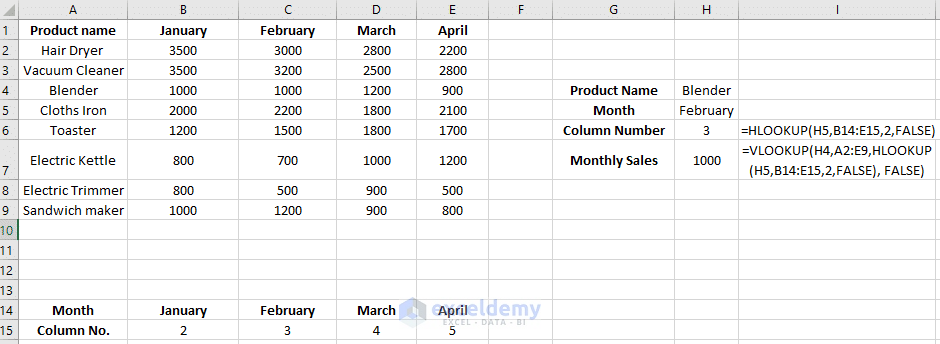Click to see the full image

## Conclusion

In this article, we saw the basics of VLOOKUP and HLOOKUP functions. The VLOOKUP and HLOOKUP combined formula was shown with an example. Instead of VLOOKUP and HLOOKUP, we can also use the INDEX and MATCH functions based on different operations. Hope you will like this article. Comment below to ask any questions and let us know your opinions on our articles.

## Related Articles

#### Siam Hasan Khan

Hello! Welcome to my Profile. Here I will be posting articles related to Microsoft Excel. I have completed my BSc in Electrical and Electronic Engineering from American International University-Bangladesh. I am a diligent, goal-oriented engineer with an immense thirst for knowledge and attitude to grow continuously. Continuous improvement and life-long learning is my motto.

George June 20, 2018 at 10:11 PM

Great instruction on how to use both VLOOKUP and HLOOKUP functions! I like this.

June 21, 2018 at 12:28 PM

vinodhini October 9, 2018 at 2:31 PM

its very good example

October 9, 2018 at 6:12 PM

Marsh December 27, 2018 at 10:33 PM

I’d love to have a few exercises to apply this knowledge in order to assimilate it properly. Thanks for considering this request.

December 29, 2018 at 3:59 PM

Hi,
You can try to use the concepts in real-world problems.
Thanks.

Anita April 5, 2019 at 10:21 PM

I can’t believe I just understood this…Appreciate the clear example, you are a great instructor!